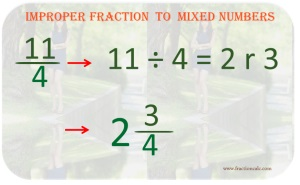## Changing Improper Fraction to Mixed number and vice versa

When doing some calculations on fractions such as addition, subtraction, multiplication, and division, there is a need to convert it to the right forms that are valid for some operation. Mixed numbers needed to be converted to improper fractions and some certain times; improper fraction will be converted to mixed numbers. This is the topic that every student should learn perfectly in order for the students to avoid mistakes in the future. Mastering the art of fraction conversion greatly helps a lot. Changing improper fraction to mixed numbers and vice versa is very helpful in the field of mathematics.

Mixed number to Improper fraction

The situation arrived when you need to add mixed numbers and a fraction. To do this, you need to convert mixed numbers to improper fraction and then apply the standard rules in adding fractions. There are easy simple steps to convert mixed number to improper fraction. Since the denominator represents the total parts of a whole and mixed numbers represent the whole parts and fractions. You need to multiply the denominator to whole number then add the numerator. The denominator is still the same. The result would be the new improper fraction. Let’s make the steps easy to grasp by creating the bulleted list below.

• Multiply the denominator to whole number and save the result.
• Add the result and the numerator. The sum is the new numerator.
• The denominator remains the same.
• With the new numerator and the same denominator, you have now the correct improper fraction.

See the photo below for the working steps applied in action.

Improper Fraction to Mixed Numbers

When people see an improper fraction, they will be having a hard time grasping the meaning of it but if you show it in mixed numbers, they will easily understand. 11/4 is always hard to understand than 2¾. So mixed numbers in essence gives clarification and simplicity of the nature of complex fractions. Mixed numbers will always be used for human readability while an improper fraction is used mainly for mathematical operations. So how do you convert improper fraction back to mixed numbers? You just divide the numerator to the denominator and the quotient will be the whole number, the remainder will be the new numerator and the denominator will remain the same. So we will make simple bulleted steps below.

• Divide the numerator to the denominator.
• The quotient will be the new whole number.
• The remainder will be the new numerator.
• The denominator will remain the same.
• You will now have the correct mixed number.

See photo below.Improper Fraction to Mixed numbers

Converting mixed numbers and improper fractions is always easy. You just need to follow every step above and you are good on your way.